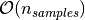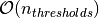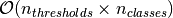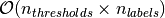Shortcuts

# Recall At Fixed Precision¶

## Module Interface¶

### BinaryRecallAtFixedPrecision¶

class torchmetrics.classification.BinaryRecallAtFixedPrecision(min_precision, thresholds=None, ignore_index=None, validate_args=True, **kwargs)[source]

Computes the highest possible recall value given the minimum precision thresholds provided. This is done by first calculating the precision-recall curve for different thresholds and the find the recall for a given precision level.

As input to forward and update the metric accepts the following input:

• preds (Tensor): A float tensor of shape (N, ...). Preds should be a tensor containing probabilities or logits for each observation. If preds has values outside [0,1] range we consider the input to be logits and will auto apply sigmoid per element.

• target (Tensor): An int tensor of shape (N, ...). Target should be a tensor containing ground truth labels, and therefore only contain {0,1} values (except if ignore_index is specified). The value 1 always encodes the positive class.

Note

Additional dimension ... will be flattened into the batch dimension.

As output to forward and compute the metric returns the following output:

• recall (Tensor): A scalar tensor with the maximum recall for the given precision level

• threshold (Tensor): A scalar tensor with the corresponding threshold level

Note

The implementation both supports calculating the metric in a non-binned but accurate version and a binned version that is less accurate but more memory efficient. Setting the thresholds argument to None will activate the non-binned version that uses memory of sizewhereas setting the thresholds argument to either an integer, list or a 1d tensor will use a binned version that uses memory of size(constant memory).

Parameters
• min_precision (float) – float value specifying minimum precision threshold.

• Can be one of:

• If set to None, will use a non-binned approach where thresholds are dynamically calculated from all the data. Most accurate but also most memory consuming approach.

• If set to an int (larger than 1), will use that number of thresholds linearly spaced from 0 to 1 as bins for the calculation.

• If set to an list of floats, will use the indicated thresholds in the list as bins for the calculation

• If set to an 1d tensor of floats, will use the indicated thresholds in the tensor as bins for the calculation.

• validate_args (bool) – bool indicating if input arguments and tensors should be validated for correctness. Set to False for faster computations.

• kwargs (Any) – Additional keyword arguments, see Advanced metric settings for more info.

Example

>>> from torchmetrics.classification import BinaryRecallAtFixedPrecision
>>> preds = torch.tensor([0, 0.5, 0.7, 0.8])
>>> target = torch.tensor([0, 1, 1, 0])
>>> metric = BinaryRecallAtFixedPrecision(min_precision=0.5, thresholds=None)
>>> metric(preds, target)
(tensor(1.), tensor(0.5000))
>>> metric = BinaryRecallAtFixedPrecision(min_precision=0.5, thresholds=5)
>>> metric(preds, target)
(tensor(1.), tensor(0.5000))


Initializes internal Module state, shared by both nn.Module and ScriptModule.

### MulticlassRecallAtFixedPrecision¶

class torchmetrics.classification.MulticlassRecallAtFixedPrecision(num_classes, min_precision, thresholds=None, ignore_index=None, validate_args=True, **kwargs)[source]

Computes the highest possible recall value given the minimum precision thresholds provided. This is done by first calculating the precision-recall curve for different thresholds and the find the recall for a given precision level.

As input to forward and update the metric accepts the following input:

• preds (Tensor): A float tensor of shape (N, C, ...). Preds should be a tensor containing probabilities or logits for each observation. If preds has values outside [0,1] range we consider the input to be logits and will auto apply softmax per sample.

• target (Tensor): An int tensor of shape (N, ...). Target should be a tensor containing ground truth labels, and therefore only contain values in the [0, n_classes-1] range (except if ignore_index is specified).

Note

Additional dimension ... will be flattened into the batch dimension.

As output to forward and compute the metric returns a tuple of either 2 tensors or 2 lists containing:

• recall (Tensor): A 1d tensor of size (n_classes, ) with the maximum recall for the given precision level per class

• threshold (Tensor): A 1d tensor of size (n_classes, ) with the corresponding threshold level per class

Note

The implementation both supports calculating the metric in a non-binned but accurate version and a binned version that is less accurate but more memory efficient. Setting the thresholds argument to None will activate the non-binned version that uses memory of sizewhereas setting the thresholds argument to either an integer, list or a 1d tensor will use a binned version that uses memory of size(constant memory).

Parameters
• num_classes (int) – Integer specifing the number of classes

• min_precision (float) – float value specifying minimum precision threshold.

• Can be one of:

• If set to None, will use a non-binned approach where thresholds are dynamically calculated from all the data. Most accurate but also most memory consuming approach.

• If set to an int (larger than 1), will use that number of thresholds linearly spaced from 0 to 1 as bins for the calculation.

• If set to an list of floats, will use the indicated thresholds in the list as bins for the calculation

• If set to an 1d tensor of floats, will use the indicated thresholds in the tensor as bins for the calculation.

• validate_args (bool) – bool indicating if input arguments and tensors should be validated for correctness. Set to False for faster computations.

• kwargs (Any) – Additional keyword arguments, see Advanced metric settings for more info.

Example

>>> from torchmetrics.classification import MulticlassRecallAtFixedPrecision
>>> preds = torch.tensor([[0.75, 0.05, 0.05, 0.05, 0.05],
...                       [0.05, 0.75, 0.05, 0.05, 0.05],
...                       [0.05, 0.05, 0.75, 0.05, 0.05],
...                       [0.05, 0.05, 0.05, 0.75, 0.05]])
>>> target = torch.tensor([0, 1, 3, 2])
>>> metric = MulticlassRecallAtFixedPrecision(num_classes=5, min_precision=0.5, thresholds=None)
>>> metric(preds, target)
(tensor([1., 1., 0., 0., 0.]), tensor([7.5000e-01, 7.5000e-01, 1.0000e+06, 1.0000e+06, 1.0000e+06]))
>>> mcrafp = MulticlassRecallAtFixedPrecision(num_classes=5, min_precision=0.5, thresholds=5)
>>> mcrafp(preds, target)
(tensor([1., 1., 0., 0., 0.]), tensor([7.5000e-01, 7.5000e-01, 1.0000e+06, 1.0000e+06, 1.0000e+06]))


Initializes internal Module state, shared by both nn.Module and ScriptModule.

### MultilabelRecallAtFixedPrecision¶

class torchmetrics.classification.MultilabelRecallAtFixedPrecision(num_labels, min_precision, thresholds=None, ignore_index=None, validate_args=True, **kwargs)[source]

Computes the highest possible recall value given the minimum precision thresholds provided. This is done by first calculating the precision-recall curve for different thresholds and the find the recall for a given precision level.

As input to forward and update the metric accepts the following input:

• preds (Tensor): A float tensor of shape (N, C, ...). Preds should be a tensor containing probabilities or logits for each observation. If preds has values outside [0,1] range we consider the input to be logits and will auto apply sigmoid per element.

• target (Tensor): An int tensor of shape (N, ...). Target should be a tensor containing ground truth labels, and therefore only contain {0,1} values (except if ignore_index is specified). The value 1 always encodes the positive class.

Note

Additional dimension ... will be flattened into the batch dimension.

As output to forward and compute the metric returns a tuple of either 2 tensors or 2 lists containing:

• recall (Tensor): A 1d tensor of size (n_classes, ) with the maximum recall for the given precision level per class

• threshold (Tensor): A 1d tensor of size (n_classes, ) with the corresponding threshold level per class

Note

The implementation both supports calculating the metric in a non-binned but accurate version and a binned version that is less accurate but more memory efficient. Setting the thresholds argument to None will activate the non-binned version that uses memory of sizewhereas setting the thresholds argument to either an integer, list or a 1d tensor will use a binned version that uses memory of size(constant memory).

Parameters
• num_labels (int) – Integer specifing the number of labels

• min_precision (float) – float value specifying minimum precision threshold.

• Can be one of:

• If set to None, will use a non-binned approach where thresholds are dynamically calculated from all the data. Most accurate but also most memory consuming approach.

• If set to an int (larger than 1), will use that number of thresholds linearly spaced from 0 to 1 as bins for the calculation.

• If set to an list of floats, will use the indicated thresholds in the list as bins for the calculation

• If set to an 1d tensor of floats, will use the indicated thresholds in the tensor as bins for the calculation.

• validate_args (bool) – bool indicating if input arguments and tensors should be validated for correctness. Set to False for faster computations.

• kwargs (Any) – Additional keyword arguments, see Advanced metric settings for more info.

Example

>>> from torchmetrics.classification import MultilabelRecallAtFixedPrecision
>>> preds = torch.tensor([[0.75, 0.05, 0.35],
...                       [0.45, 0.75, 0.05],
...                       [0.05, 0.55, 0.75],
...                       [0.05, 0.65, 0.05]])
>>> target = torch.tensor([[1, 0, 1],
...                        [0, 0, 0],
...                        [0, 1, 1],
...                        [1, 1, 1]])
>>> metric = MultilabelRecallAtFixedPrecision(num_labels=3, min_precision=0.5, thresholds=None)
>>> metric(preds, target)
(tensor([1., 1., 1.]), tensor([0.0500, 0.5500, 0.0500]))
>>> mlrafp = MultilabelRecallAtFixedPrecision(num_labels=3, min_precision=0.5, thresholds=5)
>>> mlrafp(preds, target)
(tensor([1., 1., 1.]), tensor([0.0000, 0.5000, 0.0000]))


Initializes internal Module state, shared by both nn.Module and ScriptModule.

## Functional Interface¶

### binary_recall_at_fixed_precision¶

torchmetrics.functional.classification.binary_recall_at_fixed_precision(preds, target, min_precision, thresholds=None, ignore_index=None, validate_args=True)[source]

Computes the highest possible recall value given the minimum precision thresholds provided for binary tasks. This is done by first calculating the precision-recall curve for different thresholds and the find the recall for a given precision level.

Accepts the following input tensors:

• preds (float tensor): (N, ...). Preds should be a tensor containing probabilities or logits for each observation. If preds has values outside [0,1] range we consider the input to be logits and will auto apply sigmoid per element.

• target (int tensor): (N, ...). Target should be a tensor containing ground truth labels, and therefore only contain {0,1} values (except if ignore_index is specified). The value 1 always encodes the positive class.

Additional dimension ... will be flattened into the batch dimension.

The implementation both supports calculating the metric in a non-binned but accurate version and a binned version that is less accurate but more memory efficient. Setting the thresholds argument to None will activate the non-binned version that uses memory of sizewhereas setting the thresholds argument to either an integer, list or a 1d tensor will use a binned version that uses memory of size(constant memory).

Parameters
• preds (Tensor) – Tensor with predictions

• target (Tensor) – Tensor with true labels

• min_precision (float) – float value specifying minimum precision threshold.

• Can be one of:

• If set to None, will use a non-binned approach where thresholds are dynamically calculated from all the data. Most accurate but also most memory consuming approach.

• If set to an int (larger than 1), will use that number of thresholds linearly spaced from 0 to 1 as bins for the calculation.

• If set to an list of floats, will use the indicated thresholds in the list as bins for the calculation

• If set to an 1d tensor of floats, will use the indicated thresholds in the tensor as bins for the calculation.

• validate_args (bool) – bool indicating if input arguments and tensors should be validated for correctness. Set to False for faster computations.

Returns

a tuple of 2 tensors containing:

• recall: an scalar tensor with the maximum recall for the given precision level

• threshold: an scalar tensor with the corresponding threshold level

Return type

(tuple)

Example

>>> from torchmetrics.functional.classification import binary_recall_at_fixed_precision
>>> preds = torch.tensor([0, 0.5, 0.7, 0.8])
>>> target = torch.tensor([0, 1, 1, 0])
>>> binary_recall_at_fixed_precision(preds, target, min_precision=0.5, thresholds=None)
(tensor(1.), tensor(0.5000))
>>> binary_recall_at_fixed_precision(preds, target, min_precision=0.5, thresholds=5)
(tensor(1.), tensor(0.5000))


### multiclass_recall_at_fixed_precision¶

torchmetrics.functional.classification.multiclass_recall_at_fixed_precision(preds, target, num_classes, min_precision, thresholds=None, ignore_index=None, validate_args=True)[source]

Computes the highest possible recall value given the minimum precision thresholds provided for multiclass tasks. This is done by first calculating the precision-recall curve for different thresholds and the find the recall for a given precision level.

Accepts the following input tensors:

• preds (float tensor): (N, C, ...). Preds should be a tensor containing probabilities or logits for each observation. If preds has values outside [0,1] range we consider the input to be logits and will auto apply softmax per sample.

• target (int tensor): (N, ...). Target should be a tensor containing ground truth labels, and therefore only contain values in the [0, n_classes-1] range (except if ignore_index is specified).

Additional dimension ... will be flattened into the batch dimension.

The implementation both supports calculating the metric in a non-binned but accurate version and a binned version that is less accurate but more memory efficient. Setting the thresholds argument to None will activate the non-binned version that uses memory of sizewhereas setting the thresholds argument to either an integer, list or a 1d tensor will use a binned version that uses memory of size(constant memory).

Parameters
• preds (Tensor) – Tensor with predictions

• target (Tensor) – Tensor with true labels

• num_classes (int) – Integer specifing the number of classes

• min_precision (float) – float value specifying minimum precision threshold.

• Can be one of:

• If set to None, will use a non-binned approach where thresholds are dynamically calculated from all the data. Most accurate but also most memory consuming approach.

• If set to an int (larger than 1), will use that number of thresholds linearly spaced from 0 to 1 as bins for the calculation.

• If set to an list of floats, will use the indicated thresholds in the list as bins for the calculation

• If set to an 1d tensor of floats, will use the indicated thresholds in the tensor as bins for the calculation.

• validate_args (bool) – bool indicating if input arguments and tensors should be validated for correctness. Set to False for faster computations.

Returns

a tuple of either 2 tensors or 2 lists containing

• recall: an 1d tensor of size (n_classes, ) with the maximum recall for the given precision level per class

• thresholds: an 1d tensor of size (n_classes, ) with the corresponding threshold level per class

Return type

(tuple)

Example

>>> from torchmetrics.functional.classification import multiclass_recall_at_fixed_precision
>>> preds = torch.tensor([[0.75, 0.05, 0.05, 0.05, 0.05],
...                       [0.05, 0.75, 0.05, 0.05, 0.05],
...                       [0.05, 0.05, 0.75, 0.05, 0.05],
...                       [0.05, 0.05, 0.05, 0.75, 0.05]])
>>> target = torch.tensor([0, 1, 3, 2])
>>> multiclass_recall_at_fixed_precision(preds, target, num_classes=5, min_precision=0.5, thresholds=None)
(tensor([1., 1., 0., 0., 0.]), tensor([7.5000e-01, 7.5000e-01, 1.0000e+06, 1.0000e+06, 1.0000e+06]))
>>> multiclass_recall_at_fixed_precision(preds, target, num_classes=5, min_precision=0.5, thresholds=5)
(tensor([1., 1., 0., 0., 0.]), tensor([7.5000e-01, 7.5000e-01, 1.0000e+06, 1.0000e+06, 1.0000e+06]))


### multilabel_recall_at_fixed_precision¶

torchmetrics.functional.classification.multilabel_recall_at_fixed_precision(preds, target, num_labels, min_precision, thresholds=None, ignore_index=None, validate_args=True)[source]

Computes the highest possible recall value given the minimum precision thresholds provided for multilabel tasks. This is done by first calculating the precision-recall curve for different thresholds and the find the recall for a given precision level.

Accepts the following input tensors:

• preds (float tensor): (N, C, ...). Preds should be a tensor containing probabilities or logits for each observation. If preds has values outside [0,1] range we consider the input to be logits and will auto apply sigmoid per element.

• target (int tensor): (N, C, ...). Target should be a tensor containing ground truth labels, and therefore only contain {0,1} values (except if ignore_index is specified).

Additional dimension ... will be flattened into the batch dimension.

The implementation both supports calculating the metric in a non-binned but accurate version and a binned version that is less accurate but more memory efficient. Setting the thresholds argument to None will activate the non-binned version that uses memory of sizewhereas setting the thresholds argument to either an integer, list or a 1d tensor will use a binned version that uses memory of size(constant memory).

Parameters
• preds (Tensor) – Tensor with predictions

• target (Tensor) – Tensor with true labels

• num_labels (int) – Integer specifing the number of labels

• min_precision (float) – float value specifying minimum precision threshold.

• Can be one of:

• If set to None, will use a non-binned approach where thresholds are dynamically calculated from all the data. Most accurate but also most memory consuming approach.

• If set to an int (larger than 1), will use that number of thresholds linearly spaced from 0 to 1 as bins for the calculation.

• If set to an list of floats, will use the indicated thresholds in the list as bins for the calculation

• If set to an 1d tensor of floats, will use the indicated thresholds in the tensor as bins for the calculation.

• validate_args (bool) – bool indicating if input arguments and tensors should be validated for correctness. Set to False for faster computations.

Returns

a tuple of either 2 tensors or 2 lists containing

• recall: an 1d tensor of size (n_classes, ) with the maximum recall for the given precision level per class

• thresholds: an 1d tensor of size (n_classes, ) with the corresponding threshold level per class

Return type

(tuple)

Example

>>> from torchmetrics.functional.classification import multilabel_recall_at_fixed_precision
>>> preds = torch.tensor([[0.75, 0.05, 0.35],
...                       [0.45, 0.75, 0.05],
...                       [0.05, 0.55, 0.75],
...                       [0.05, 0.65, 0.05]])
>>> target = torch.tensor([[1, 0, 1],
...                        [0, 0, 0],
...                        [0, 1, 1],
...                        [1, 1, 1]])
>>> multilabel_recall_at_fixed_precision(preds, target, num_labels=3, min_precision=0.5, thresholds=None)
(tensor([1., 1., 1.]), tensor([0.0500, 0.5500, 0.0500]))
>>> multilabel_recall_at_fixed_precision(preds, target, num_labels=3, min_precision=0.5, thresholds=5)
(tensor([1., 1., 1.]), tensor([0.0000, 0.5000, 0.0000]))


© Copyright Copyright (c) 2020-2023, Lightning-AI et al... Revision 825d17f3.

Built with Sphinx using a theme provided by Read the Docs.
Versions
latest
stable
v0.11.3
v0.11.2
v0.11.1
v0.11.0
v0.10.3
v0.10.2
v0.10.1
v0.10.0
v0.9.3
v0.9.2
v0.9.1
v0.9.0
v0.8.2
v0.8.1
v0.8.0
v0.7.3
v0.7.2
v0.7.1
v0.7.0
v0.6.2
v0.6.1
v0.6.0
v0.5.1
v0.5.0
v0.4.1
v0.4.0
v0.3.2
v0.3.1
v0.3.0
v0.2.0
v0.1.0